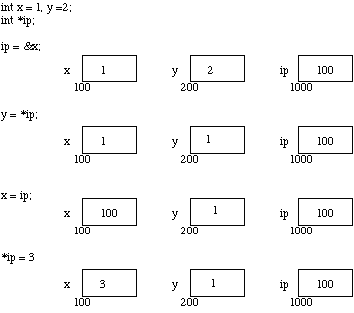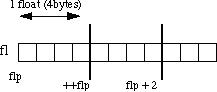# Pointers

Pointer

A pointer is a variable which contains the address in memory of another variable. We can have a pointer to any variable type.

The & operator gives the address of the variable pointed by the pointer. The indirection or de-reference operator * gives the contents of the variable pointed to by a pointer.

To declare a pointer to a variable do:

Data-type *pointer_variable_name;

NOTE: We must associate a pointer to a particular type: You can’t assign the address of a short int to a long int, for instance.

Consider the effect of the following code:

int x = 1, y = 2;

int *ip;

ip = &x;

y = *ip;

x = ip;

*ip = 3;

Assume that the variable x resides at memory location 100, y at 200 and ip at 1000.The assignments x = 1 and y = 2 load these values into the variables. ip is declared to be a pointer to an integer and is assigned to the address of x (&x). So ip gets loaded with the value 100.

Next y gets assigned to the contents of ip. In this example ip currently points to memory location 100 ,the location of x. So y gets assigned to the values of x, which is 1.

It is legal to assign the current value of ip to x. The value of ip at this instant is 100.Finally we can assign a value to the contents of a pointer (*ip).

IMPORTANT: When a pointer is declared it does not point anywhere. You must set it to point somewhere before you use it.

So …

int *ip;

*ip = 100;

will generate an error

The correct use is:

int *ip;

int x;

ip = &x;

*ip = 100; // Now the value of x is 100

We can do integer arithmetic on a pointer:

int *ip;

int i=10;

ip= &i;

*ip = *ip + 5;

++*ip;

(*ip)++;

// The value of i and *ip would be 17

The reason we associate a pointer to a data type is so that it knows how many bytes the data is stored in. When we increment a pointer we increase the pointer by one block  memory.

Consider a float variable (fl) and a pointer to a float (flp)Pointer Arithmetic Assume that flp points to fl then if we increment the pointer ( ++flp) it moves to the position shown 4 bytes on. If on the other hand we added 2 to the pointer then it moves 2 float positions i.e 8 bytes as shown in the Figure.

Dynamic memory allocation/deallocation operators

// Use of new and delete operators
#include <iostream.h>

int main ()
{
int *intp = NULL; // Pointer initialized with null
intp = new int;   // Request memory for the variable

*intp = 123;      // Store value at allocated address
cout << “Pointer intp = ” << *intp << endl;

delete intp ;     // free up the memory.

return 0;
}

Reference variable

A reference variable is an alias, that is, another name for an already existing variable. Once a reference is initialized with a variable, either the variable name or the reference name may be used to refer to the variable.

Reference vs Pointer variable:

References are often confused with pointers but three major differences between references and pointers are:

1. You cannot have NULL reference for a reference variable
2. Once a reference variable is initialized to an object, it cannot be changed to refer to another object. Pointers can be pointed to another object at any time.
3. A reference must be initialized when it is created. Pointers can be initialized at any time.

// Reference variable Example

#include <iostream.h>
int main ()
{
// declare simple variables
int    i;

// declare reference variables
int &r = i; // Now we can refer i as r or r is a alias for i
i = 5;
cout << “Value of i = ” << i << endl;
cout << “Value of reference variable r = ” << r  << endl;
return 0;
}

Output
Value of i = 5
Value of reference variable r = 5
Call by value and Call by Reference

When C++ passes arguments to functions it passes them by value. There are many cases when we may want to alter a passed argument in the function and receive the new value back to the calling function. C++ uses pointers explicitly to do this. The best way to study this is to look at an example where we must be able to receive changed parameters. Let us try and write a function to swap variables:

// Call by Value Example
#include<iostream.h>
int main()

{
void swap(int m,int m);
int a=10 ,b=20;
swap(a,b); // Call by value
cout<<“After interchanging the values “;

cout<<” a = “<<a<<endl<<“b = “<<b;
return 0;
}
void swap(int m ,int n)

{
int a;
a=m;
m=n;
n=a;
cout<<“Inside swap function the values are “;
cout<<endl<<” m = “<<m<<endl<<” n= “<<n;
}
output:
Inside swap function the values are
m=20
n=10;
After interchanging the values
a=10
b=20

Note: No change to the values of a and b inside the calling function .Because when we pass values to the function only the copies of those values get passed not the original data value .Any change made inside the called function to these data value is not reflected to the calling function. That is why we call it call by value.
swap(a, b)   WON’T WORK.

Pointers provide the solution: Pass the address of the variables to the functions and access address of function.

Thus our function call in our program would look like this:

swap(&a, &b)

// Call by Reference Example

#include<iostream.h>
int main()

{

void swap(int *m,int *m);

int a=10 ,b=20;

swap(&a,&b); // Call by reference

cout<<“After interchanging the values “;

cout<<” a = “<<a<<endl<<“b = “<<b;

return 0;

}
void swap(int *m ,int *n)

{
int a;

a=*m;

*m=*n;

*n=a;

cout<<“Inside swap function the values are “;

cout<<endl<<” *m = “<<m<<endl<<” *n= “<<n;

}
output:
Inside swap function the values are

*m=20
*n=10;
After interchanging the values

a=20
b=10

Note: The values of a and b changed .Because when we pass reference of the values to the function  original data value are passed .Any change made inside the called function to these data value is reflected to the calling function. That is why we call it call by Reference.

Let us see another way of call by reference using C++ reference variable .

// Using Reference variables method

#include<iostream.h>
int main()

{
void swap(int &m,int &m);
int a=10 ,b=20;
cout<<“After interchanging the values “;
swap(a,b); // Call by value
cout<<” a = “<<a<<endl<<“b = “<<b;
return 0;
}
void swap(int &m ,int &n)// use of reference variable

{
int a;
a=m;
m=n;
n=a;
cout<<“Inside swap function the values are “;
cout<<endl<<” m = “<<m<<endl<<” n= “<<n;
}
output:
Inside swap function the values are
m=20
n=10;
After interchanging the values
a=20
b=10

Pointers and Arrays

Pointers and arrays are very closely linked.

Consider the following:

int a, x;
int *pa;
pa = &a; /* pa pointer to address of a */
x = *pa;
/* x = contents of pa (a in this case) */

Arrays and Pointers

To get somewhere in the array using a pointer we could do:

(pa + i)= a[i]

C++ however is much more subtle in its link between arrays and pointers.

For example we can just type

pa = a;

pa = &a

and

a[i] can be written as *(a + i)

We also express pointer addressing like this:

pa[i] = *(pa + i)

However pointers and arrays are different:

A pointer is a variable. We can do

pa = a;
pa++ ;

An Array is not a variable.

a = pa and a++ ARE ILLEGAL.

We can now understand how arrays are passed to functions. When an array is passed to a function what is actually passed is its initial elements location in memory.

So:

int a[]={1,2};

void arpass(int a[]);

arpass(a) ;

/* Passing array in a function. Here only name of the array is required, it is same as arpass(&a);  */

Example of passing array in a function

#include <iostream.h>

int main ()
{

int a[]={1,2}; // Declare simple int arry
void arpass(int a[]); // Function declaration for accepting array as an argument

arpass(a); //At the time of call only the name of the array needs to specified

return 0;
}
void arpass(int a[]) // Function Definition
{
for(int i=0;i<2;i++)
cout<<a[i];
}

Pointer to an One Dimensional Array

#include <iostream.h>

int main ()
{
int i,a;
int *p;
p=a;    // same as p = &a
cout<<endl<<“Enter 5 Numbers “;

for(i=0;i<5;i++)
cin>>p[i];    // same as a[i]
//Other form of the above statement is cin>>*(p+i);

cout<<endl<<“You have Entered”;

for(i=0;i<5;i++)
cout<<p[i]<<endl;   // same as a[i]
// Other form of the above statement is cout<<*(p+i);
return 0;
}

Function Returning a Pointer

If a function return type is a Pointer of any data type then we say that this function will return Pointer.

// A c++  Example of Function  returning Pointer
#include<iostream.h>
int main()
{
int *func(int a); // this function will return a Pointer
int *p;
int a;
cout<<“Enter a Number”;
cin>>a;
p=func(a); // P will point to the pointer returned by *func()
cout<<” *p  = “<<*p;
return 0;
}
int *func(int a)
{
int *t;
t =&a;
return t; // This statement will return a Pointer
}

Arrays of Pointers

There may be a situation when we want to maintain an array which can store pointers to an int or char or any other data type available. Following is the declaration of an array of pointers to character:

int *ptr[SIZE];

This declares ptr as an array of SIZE integer pointers. Thus, each element in ptr, now holds a pointer to an integer value. Following example makes use of two integer pointers which will be stored in an array of pointers as follows:

Array of Pointer Example:

#include <iostream.h>
const int SIZE = 2;
int main ()
{
int i=10,j=20 ,*ip ,*jp;

int *arrp[SIZE];

ip=&i;

jp=&j;

arrp=ip;

arrp=jp;

for (int t = 0; t < SIZE; t++)
{
cout << “Value of arrp[” << t << “] = “;
cout << *arrp[i] << endl;
}
return 0;
}
When the above code is compiled and executed, it produces following result:
Value of arrp = 10
Value of arrp = 20

Pointers and Structures

These are fairly straight forward and are easily defined. Consider the following:

struct Ayan

{

int a,b;

}ap;

struct Ayan  *aptr;

aptr = &ap; // assigns pointer to &ap

the -> operator lets us access a member of the structure pointed to by a pointer i.e.:

aptr->a = 10;

aptr->b = 20;

Example of Pointers and Structure

#include <iostream.h>

struct Ayan

{
int a,b;
}ap; // Structure variable
struct Ayan *aptr; // Pointer Structure variable

int main()

{
aptr = &ap; // Assigns address of ap to pointer aptr

// Accessing structure members through Pointer

aptr->a=10;

aptr->b=20;
cout<<endl<<“a =” <<aptr->a<<” b = “<<aptr->b;

return 0;

}

Output

a=10

b=20;

Self Referential Structure

Suppose we have a structure declaration linke this

struct list

{

int data;

struct list *next;

//Above statement makes struct list as self referential

}*node;

Any structure which have  a member of type structure itself is called a Self referential structure. In the above example structure list contain a member *next whose data type is structure itself, this will makes struct list as Self Referential Structure. These types of structures are generally used for Queue,Stack and Linked List implementation.